Nonlin. Processes Geophys., 27, 373–389, 2020
https://doi.org/10.5194/npg-27-373-2020
Nonlin. Processes Geophys., 27, 373–389, 2020
https://doi.org/10.5194/npg-27-373-2020

Research article 02 Jul 2020

Research article | 02 Jul 2020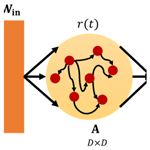# Data-driven predictions of a multiscale Lorenz 96 chaotic system using machine-learning methods: reservoir computing, artificial neural network, and long short-term memory network

Data-driven predictions of a multiscale Lorenz 96 chaotic system using machine-learning methods: reservoir computing, artificial neural network, and long short-term memory network
Ashesh Chattopadhyay1, Pedram Hassanzadeh1,2, and Devika Subramanian3,4 Ashesh Chattopadhyay et al.
• 1Department of Mechanical Engineering, Rice University, Houston, TX, USA
• 2Department of Earth Environmental and Planetary Sciences, Rice University, Houston, TX, USA
• 3Department of Electrical and Computer Engineering, Rice University, Houston, TX, USA
• 4Department of Computer Science, Rice University, Houston, TX, USA

Correspondence: Pedram Hassanzadeh (pedram@rice.edu)

Abstract

In this paper, the performance of three machine-learning methods for predicting short-term evolution and for reproducing the long-term statistics of a multiscale spatiotemporal Lorenz 96 system is examined. The methods are an echo state network (ESN, which is a type of reservoir computing; hereafter RC–ESN), a deep feed-forward artificial neural network (ANN), and a recurrent neural network (RNN) with long short-term memory (LSTM; hereafter RNN–LSTM). This Lorenz 96 system has three tiers of nonlinearly interacting variables representing slow/large-scale (X), intermediate (Y), and fast/small-scale (Z) processes. For training or testing, only X is available; Y and Z are never known or used. We show that RC–ESN substantially outperforms ANN and RNN–LSTM for short-term predictions, e.g., accurately forecasting the chaotic trajectories for hundreds of numerical solver's time steps equivalent to several Lyapunov timescales. The RNN–LSTM outperforms ANN, and both methods show some prediction skills too. Furthermore, even after losing the trajectory, data predicted by RC–ESN and RNN–LSTM have probability density functions (pdf's) that closely match the true pdf – even at the tails. The pdf of the data predicted using ANN, however, deviates from the true pdf. Implications, caveats, and applications to data-driven and data-assisted surrogate modeling of complex nonlinear dynamical systems, such as weather and climate, are discussed.

Share
1 Introduction

Various components of the Earth system involve multiscale, multiphysics processes and high-dimensional chaotic dynamics. These processes are often modeled using sets of strongly coupled nonlinear partial differential equations (PDEs), which are solved numerically on supercomputers. As we aim to simulate such systems with increasing levels of fidelity, we need to increase the numerical resolutions and/or incorporate more physical processes from a wide range of spatiotemporal scales into the models. For example, in atmospheric modeling for predicting the weather and climate systems, we need to account for the nonlinear interactions across the scales of cloud microphysics processes, gravity waves, convection, baroclinic waves, synoptic eddies, and large-scale circulation, just to name a few (not to mention the fast/slow processes involved in feedbacks from the ocean, land, and cryosphere; Collins et al.2006, 2011; Flato2011; Bauer et al.2015; Jeevanjee et al.2017).

Solving the coupled systems of PDEs representing such multiscale processes is computationally highly challenging for many practical applications. As a result, to make the simulations feasible, a few strategies have been developed, which mainly involve only solving for slow/large-scale variables and accounting for the fast/small-scale processes through surrogate models. In weather and climate models, for example, semiempirical physics-based parameterizations are often used to represent the effects of processes such as gravity waves and moist convection in the atmosphere or submesoscale eddies in the ocean . A more advanced approach is “super-parameterization” which, for example, involves solving the PDEs of moist convection on a high-resolution grid inside each grid point of large-scale atmospheric circulation for which the governing PDEs (the Navier–Stokes equations) are solved on a coarse grid . While computationally more expensive, super-parameterized climate models have been shown to outperform parameterized models in simulating some aspects of climate variability and extremes .

More recently, “inexact computing” has received attention from the weather and climate community. This approach involves reducing the computational cost of each simulation by decreasing the precision of some of the less-critical calculations (Palem2014; Palmer2014), thus allowing the saved resources to be reinvested, for example, in more simulations (e.g., for probabilistic predictions) and/or higher resolutions for critical processes . One type of inexact computing involves solving the PDEs of some of the processes with single- or half-precision arithmetic, which requires less computing power and memory compared to the conventional double-precision calculations .

In the past few years, the rapid algorithmic advances in machine learning (ML) and in particular data-driven modeling have been explored for improving the simulations and predictions of nonlinear dynamical systems . One appeal of the data-driven approach is that fast and accurate data-driven surrogate models that are trained on data of high fidelity can be used to accelerate and/or improve the predictions and simulations of complex dynamical systems. Furthermore, for some poorly understood processes for which observational data are available (e.g., clouds), data-driven surrogate models built using such data might potentially outperform physics-based surrogate models . Recent studies have shown promising results in using ML to build data-driven parameterizations for the modeling of some atmospheric and oceanic processes . In the turbulence and dynamical systems communities, similarly encouraging outcomes have been reported .

The objective of the current study is to make progress toward addressing the following question: which AI-based data-driven technique(s) can best predict the spatiotemporal evolution of a multiscale chaotic system, when only the slow/large-scale variables are known (during training) and are of interest (to predict during testing)? We emphasize that, unlike many other studies, our focus is not on reproducing long-term statistics of the underlying dynamical system (although that will be examined too) but on predicting the short-term trajectory from a given initial condition. Furthermore, we emphasize that, following the problem formulation introduced by , the system's state vector is only partially known and the fast/small-scale variables are unknown even during training.

Our objective is more clearly demonstrated using the canonical chaotic system that we will use as a test bed for the data-driven methods, a multiscale Lorenz 96 system, as follows:

$\begin{array}{ll}\text{(1)}& \frac{\mathrm{d}{X}_{k}}{\mathrm{d}t}=& \phantom{\rule{0.125em}{0ex}}{X}_{k-\mathrm{1}}\left({X}_{k+\mathrm{1}}-{X}_{k-\mathrm{2}}\right)+F-\frac{hc}{b}{\mathrm{\Sigma }}_{j}{Y}_{j,k},\frac{\mathrm{d}{Y}_{j,k}}{\mathrm{d}t}=& \phantom{\rule{0.125em}{0ex}}-cb{Y}_{j+\mathrm{1},k}\left({Y}_{j+\mathrm{2},k}-{Y}_{j-\mathrm{1},k}\right)-c{Y}_{j,k}+\frac{hc}{b}{X}_{k}\\ \text{(2)}& & \phantom{\rule{0.125em}{0ex}}-\frac{he}{d}{\mathrm{\Sigma }}_{i}{Z}_{i,j,k},\frac{\mathrm{d}{Z}_{i,j,k}}{\mathrm{d}t}=& \phantom{\rule{0.125em}{0ex}}ed{Z}_{i-\mathrm{1},j,k}\left({Z}_{i+\mathrm{1},j,k}-{Z}_{i-\mathrm{2},j,k}\right)-ge{Z}_{i,j,k}\\ \text{(3)}& & +\frac{he}{d}{Y}_{j,k}.\end{array}$

This set of coupled nonlinear ordinary differential equations (ODEs) is a three-tier extension of Lorenz's original model (Lorenz1996) and has been proposed by as a fitting prototype for multiscale chaotic variability of the weather and climate system and a useful test bed for novel methods. In these equations, F=20 is a large-scale forcing that makes the system highly chaotic, and $b=c=e=d=g=\mathrm{10}$ and h=1 are tuned to produce appropriate spatiotemporal variability of the three variables (see below). The indices are $i,j,k=\mathrm{1},\mathrm{2},\mathrm{\dots }\mathrm{8}$; thus X has 8 elements while Y and Z have 64 and 512 elements, respectively. Figure 1 shows examples of the chaotic temporal evolution of X, Y, and Z obtained from directly solving Eqs. (1)–(3). The examples demonstrate that X has large amplitudes and slow variability; Y has relatively small amplitudes, high-frequency variability, and intermittency; and Z has small amplitudes and high-frequency variability. In the context of atmospheric circulation, the slow variable X can represent the low-frequency variability of the large-scale circulation, while the intermediate variable Y and fast variable Z can represent synoptic and baroclinic eddies and small-scale convection, respectively. Similar analogies in the context of ocean circulation and various other natural or engineering systems can be found, making this multiscale Lorenz 96 system a useful prototype to focus on.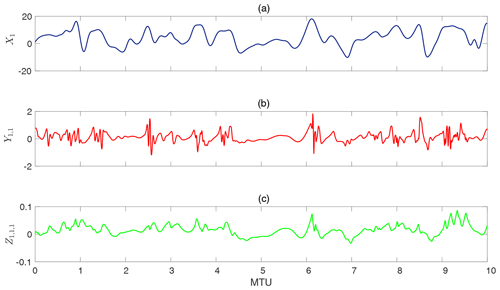Figure 1Time evolution of (a) Xk at grid point k=1, (b) Yj,1 at grid point j=1, and (c) ${Z}_{i,\mathrm{1},\mathrm{1}}$ at grid point i=1. The time series show chaotic behavior in X, which has large amplitude and slow variability; Y, which has relatively small amplitudes, high-frequency variability, and intermittency; and Z, which has small amplitudes and high-frequency variability. The x axis is in model time units (MTUs), which are related to the time step of the numerical solver t) and largest positive Lyapunov exponent (λmax) as $\mathrm{1}\phantom{\rule{0.125em}{0ex}}\mathrm{MTU}=\mathrm{200}\mathrm{\Delta }t\approx \mathrm{4.5}/{\mathit{\lambda }}_{max}$ (see Sect. 2.1). Note that here we are presenting raw data which have not been standardized for predictions or testing yet.

Our objective is to predict the spatiotemporal evolution of X(t) using a data-driven model that is trained on past observations of X(t); Fig. 2. In the conventional approach of solving Eqs. (1)–(3) numerically, the governing equations have to be known, initial conditions for Y(t) and Z(t) have to be available, and the numerical resolution is dictated by the fast/small-scale variable Z, leading to high computational costs. In the fully data-driven approach that is our objective here, the governing equations do not have to be known, Y(t) and Z(t) do not have to be observed at any time, and the evolution of X(t) is predicted just from knowledge of the past observations of X, leading to low computational costs. To successfully achieve this objective, a data-driven method should be able to do the following:

1. accurately predict the evolution of a chaotic system along a trajectory for some time

2. account for the effects of Y(t) and Z(t) on the evolution of X(t).

Inspired by several recent studies (which will be discussed below), we have focused on evaluating the performance of three ML techniques in accomplishing (1) and (2). These data-driven methods are as follows:

• RC–ESN: echo state network (ESN), a specialized type of recurrent neural network (RNN), which belongs to the family of reservoir computing (RC);

• ANN: a deep feed-forward artificial neural network; and

• RNN–LSTM: an RNN with long short-term memory (LSTM).

We have focused on these three methods because they have either shown promising performance in past studies (RC–ESN and ANN), or they are considered to be state of the art in learning from sequential data (RNN–LSTM). There are a growing number of studies focused on using machine-learning techniques for data-driven modeling of chaotic and turbulent systems, for example, to improve weather and climate modeling and predictions. Some of these studies have been referenced above. Below, we briefly describe three sets of studies with the closest relevance to our objective and approach. Pathak and coworkers have recently shown promising results with RC–ESN for predicting short-term spatiotemporal evolution (item 1) and in replicating attractors for the Lorenz 63 and Kuramoto–Sivashinsky equations. The objective of our paper (items 1–2) and the employed multiscale Lorenz 96 system are identical to that of , who reported their ANN to have some skills in data-driven predictions of the spatiotemporal evolution of X. Finally, found an RNN–LSTM to have skills (though limited) for predicting the short-term spatiotemporal evolution (item 1) of a number of chaotic toy models such as the original Lorenz 96 system. Here we aim to build on these pioneering studies and examine, side by side, the performance of RC–ESN, ANN, and RNN–LSTM in achieving (1) and (2) for the chaotic multiscale Lorenz 96 system. We emphasize the need for a side-by-side comparison that uses the same system as the test bed and metrics for assessing performance.

The structure of the paper is as follows: in Sect. 2, the multiscale Lorenz 96 system and the three ML methods are discussed, results on how these methods predict the short-term spatiotemporal evolution of X and reproduce the long-term statistics of X are presented in Sect. 3, and key findings and future work are discussed in Sect. 4.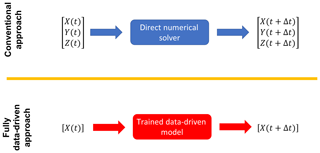Figure 2The conventional approach of solving the full governing equations numerically versus our data-driven approach for predicting the spatiotemporal evolution of the slow/large-scale variable X. For the direct numerical solution, the governing equations have to be known and the numerical resolution is dictated by the fast and small-scale variable Z, resulting in high computational costs. In the data-driven approach, the governing equations do not have to be known, Y and Z do not have to be known, and evolution of X is predicted just from knowing the past observations of X, leading to low computational costs.

2 Materials and methods

## 2.1 The multiscale Lorenz 96 system

### 2.1.1 Numerical solution

We have used a fourth-order Runge–Kutta solver, with time step Δt=0.005, to solve the system of Eqs. (1)–(3). The system has been integrated for 100 million time steps to generate a large dataset for training, testing, and examination of the robustness of the results. In the Results section, we often report time in terms of model time units (MTUs), where 1 MTU=200Δt. In terms of the Lyapunov timescale, 1 MTU in this system is $\approx \mathrm{4.5}/{\mathit{\lambda }}_{max}$ , where λmax is the largest positive Lyapunov exponent. In terms of the e-folding decorrelation timescale (τ) of the leading principal component (PC1) of X , we estimate 1 MTU≈6.9τPC1. Finally, as discussed in , 1 MTU in this system corresponds to ≈3.75 Earth days in the real atmosphere.

### 2.1.2 Training and testing datasets

To build the training and testing datasets from the numerical solution, we have sampled X∈ℜ8 at every Δt and then standardized the data by subtracting the mean and dividing by the standard deviation. We have constructed a training set consisting of N sequential samples and a testing set consisting of the next 2000 sequential samples from point N+1 to N+2000. We have randomly chosen 100 such training/testing sets, each of length (N+2000)Δt, starting from a random point and separated from the next training/testing set by at least 2000Δt. There is never any overlap between the training and the testing sequences in any of the 100 training/testing sets.

## 2.2 Reservoir computing–echo state network (RC–ESN)

### 2.2.1 Architecture

The RC–ESN is an RNN that has a reservoir with D neurons, which are sparsely connected in an Erdős–Rényi graph configuration (see Fig. 3). The connectivity of the reservoir neurons is represented by the adjacency matrix A of size D×D for which the values are drawn from a uniform random distribution on the interval $\left[-\mathrm{1},\mathrm{1}\right]$. The adjacency matrix A is then normalized by its maximum eigenvalue (Λmax) and then further multiplied with a scalar (ρ≤1) to build the $\stackrel{\mathrm{̃}}{\mathbf{A}}$ matrix. The state of the reservoir, representing the activations of its constituent neurons, is a vector r(t)∈ℜD. The typical reservoir size used in this study is D=5000 (but we have experimented with D as large as 20 000, as discussed later). The other two components of the RC–ESN are an input-to-reservoir layer, with a weight matrix of Win, and a reservoir-to-output layer, with a weight matrix of Wout. The inputs for training are N sequential samples of X(t)∈ℜ8. At the beginning of the training phase, A and Win are chosen randomly and are fixed; i.e., they do not change during training or testing and $\stackrel{\mathrm{̃}}{\mathbf{A}}$ is calculated. During training, only the weights of the output-to-reservoir layer (Wout) are updated.

Wout is the only trainable matrix in this network. This architecture yields a simple training process that has the following two key advantages:

• It does not suffer from the vanishing and the exploding gradient problem, which has been a major difficulty in training RNNs, especially before the advent of LSTMs .

• Wout can be computed in one shot (see below); thus this algorithm is orders of magnitude faster than the backpropagation through time (BPTT) algorithm , which is used for training general RNNs and RNN–LSTMs (see Sect. 2.4).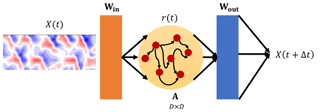Figure 3A schematic of RC–ESN. D×D is the size of the adjacency matrix of reservoir A. X(t) ($-T\le t\le \mathrm{0}$) is the input during training. Win and A are random matrices that are chosen at the beginning of training and fixed during training and testing. Only Wout is computed during training. During testing (t>0), X(tt) is predicted from a given X(t) that is either known from the initial condition or has been previously predicted.

The equations governing the RC–ESN training process are as follows:

$\begin{array}{}\text{(4)}& & r\left(t+\mathrm{\Delta }t\right)=\mathrm{tanh}\left(\stackrel{\mathrm{̃}}{\mathbf{A}}r\left(t\right)+{\mathbf{W}}_{\text{in}}X\left(t\right)\right),\text{(5)}& & {\mathbf{W}}_{\text{out}}=\mathrm{arg}\underset{{\mathbf{W}}_{\text{out}}}{min}\parallel {\mathbf{W}}_{\text{out}}\stackrel{\mathrm{̃}}{r}\left(t\right)-X\left(t\right)\parallel +\mathit{\alpha }\parallel {\mathbf{W}}_{\text{out}}\parallel .\end{array}$

Here $\parallel \cdot \parallel$ is the L2-norm of a vector and α is the L2 regularization (ridge regression) constant. Equation (4) maps the observable X(t)∈ℜ8 to the higher-dimensional reservoir's state $r\left(t+\mathrm{\Delta }t\right)\in {\mathrm{\Re }}^{D}$ (reminder: D is O(1000) for this problem). Note that Eq. (5) contains $\stackrel{\mathrm{̃}}{r}\left(t\right)$ rather than r(t). As observed by for the Kuramoto–Sivashinsky equation, and observed by us in this work, the columns of the matrix $\stackrel{\mathrm{̃}}{\mathbf{r}}$ should be chosen as nonlinear combinations of the columns of the reservoir state matrix r (each row of the matrix r is a snapshot r(t) of size D). For example, following , we compute $\stackrel{\mathrm{̃}}{\mathbf{r}}$ as having the same even columns as r, while its odd columns are the square of the odd columns of r (algorithm T1 hereafter). As shown in Appendix A, we have found that a nonlinear transformation (between r and $\stackrel{\mathrm{̃}}{\mathbf{r}}$) is essential for skillful predictions, while several other transformation algorithms yield similar results to T1. The choices of these transformations (T2 and T3; see Appendix A), although not based on a rigorous mathematical analysis, are inspired from the nature of the quadratic nonlinearity that is present in Eqs. (1)–(3).

The prediction process is governed by the following:

$\begin{array}{}\text{(6)}& X\left(t+\mathrm{\Delta }t\right)={\mathbf{W}}_{\text{out}}\stackrel{\mathrm{̃}}{r}\left(t+\mathrm{\Delta }t\right),\end{array}$

where $\stackrel{\mathrm{̃}}{r}\left(t+\mathrm{\Delta }t\right)$ in Eq. (6) is computed by applying one of the T1, T2, or T3 algorithms on r(tt), which itself is calculated via Eq. (4) from X(t) that is either known from the initial condition or has been previously predicted.

See , , , and references therein for further discussions on RC–ESNs, and , , , , , , , , for examples of recent applications to dynamical systems.

### 2.2.2 Training and predictions

During training ($-T\le t\le \mathrm{0}$), Win and A are initialized with random numbers, which stay fixed during the training (and testing) process. The state matrix r is initialized to 0. For all the experiments conducted in this study, we have empirically found that the value of ρ=0.1 yields the best results in our experiments. We have further found that in $\mathrm{0.05}\le \mathit{\rho }\le \mathrm{0.7}$ the prediction horizon of RC–ESN is not sensitive to ρ; see Appendix B. Then, the state matrix r is computed using Eq. (4) for the training set, and Wout is computed using Eq. (5). During predictions (i.e., testing) corresponding to t>0, the computed Wout is used to march forward in time (Eq. 6) while as mentioned earlier, r(t) keeps being updated using the predicted X(t); see Eq. 4. A nonlinear transformation is used to compute $\stackrel{\mathrm{̃}}{r}\left(t\right)$ from r(t) before using Eq. (6).

Our RC–ESN architecture and training/prediction procedure are similar to the ones used in . There is no overfitting in the training phase because the final training and testing accuracies are the same. Our code is developed in Python and is made publicly available (see Code and data availability).

## 2.3 Feed-forward artificial neural network (ANN)

### 2.3.1 Architecture

We have developed a deep ANN that has the same architecture as the one used in (which they employed to conduct predictions on the same multiscale Lorenz 96 studied here). The ANN has four hidden layers with 100 neurons each and 8 neurons in the input and output layers (Fig. 4). It should be noted that, unlike RC–ESN (and RNN–LSTM), this ANN is stateless, i.e., there is no hidden variable such as r(t) that tracks temporal evolution. Furthermore, unlike RC–ESN, for which the input and output are X(t), and X(tt), following , the ANN's inputs/outputs are chosen to be pairs of X(t)train and $\mathrm{\Delta }X\left(t{\right)}^{\text{train}}=X\left(t+\mathrm{\Delta }t{\right)}^{\text{train}}-X\left(t{\right)}^{\text{train}}$ (Fig. 4). During the prediction, using X(t)test that is either known from the initial condition or has been previously calculated, ΔX(t)test is predicted. X(tt)test is then computed via the Adams–Bashforth integration scheme as follows:

$\begin{array}{}\text{(7)}& X\left(t+\mathrm{\Delta }t{\right)}^{\text{test}}=X\left(t{\right)}^{\text{test}}+\frac{\mathrm{1}}{\mathrm{2}}\left[\mathrm{3}\mathrm{\Delta }X\left(t{\right)}^{\text{test}}-\mathrm{\Delta }X\left(t-\mathrm{\Delta }t{\right)}^{\text{test}}\right].\end{array}$

More details on the ANN architecture have been reported in Appendix C.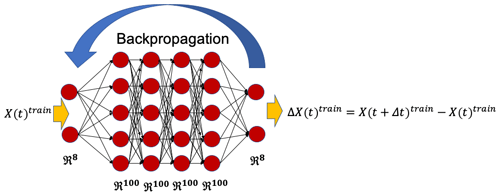Figure 4A schematic of ANN, which has four hidden layers each with 100 neurons and an input and an output layer each with 8 neurons (for better illustration, only a few neurons from each layer are shown). Training is performed on pairs of X(t)train and ΔX(t)train, and the weights in each layer are learned through the backpropagation algorithm. During testing (i.e., predictions) ΔX(t)test is predicted for a given X(t)test that is either known from the initial condition or that has been previously predicted. Knowing X(t)test, ΔX(t)test, and ΔX(t−Δt)test, X(tt)test is then computed using the Adams–Bashforth integration scheme.

### 2.3.2 Training and predictions

Training is performed on N pairs of sequential (X(t)trainX(t)train) from the training set. During training, the weights of the network are computed using backpropagation optimized by the stochastic gradient descent algorithm. During predictions, as mentioned above, ΔX(t)test is predicted from X(t)test that is either known from the initial condition or has been previously predicted, and X(tt)test is then calculated using Eq. (7).

Our ANN architecture and training/prediction procedure are similar to the ones used in . We have optimized, by trial and error, the hyperparameters for this particular network. There is no overfitting in the training phase because the final training and testing accuracies are the same. Our code is developed in Keras and is made publicly available (see Code and data availability).

## 2.4 Recurrent neural network with long short-term memory (RNN–LSTM)

### 2.4.1 Architecture

The RNN–LSTM is a deep-learning algorithm most suited for the predictions of sequential data, such as time series, and has received a lot of attention in recent years . Variants of RNN–LSTMs are the best-performing models for time series modeling in areas such as stock pricing , supply chain , natural language processing , and speech recognition . A major improvement over regular RNNs, which have issues with the vanishing and the exploding gradients , LSTMs have become the state-of-the-art approach for training RNNs in the deep-learning community. Unlike regular RNNs, the RNN–LSTMs have gates that control the information flow into the neural network from previous time steps of the time series. The RNN–LSTM used here, like the RC–ESN but unlike the ANN, is stateful (i.e., actively maintains its state). Our RNN–LSTM has 50 hidden layers in each RNN–LSTM cell. More details on our RNN–LSTM are presented in Appendix D. RNN–LSTMs have many tunable parameters and are trained with the expensive BPTT algorithm .

### 2.4.2 Training and predictions

The input to the RNN–LSTM is a time-delay-embedded matrix of X(t) with embedding dimension q (also known as lookback; Kim et al.1999). An extensive hyperparameter optimization (by trial and error) is performed to find the optimal value of q for which the network has the largest prediction horizon (exploring $q=\mathrm{1}-\mathrm{22}$, we found q=3 to yield the best performance). The RNN–LSTM predicts X(tt) from the previous q time steps of X(t). This is in contrast with RC–ESN, which only uses X(t) and reservoir state r(t) to predict X(tt), and ANN, which only uses X(t) and no state to predict X(tt) (via predicting ΔX(t)). The weights of the LSTM layers are determined during the training process (see Appendix D). During testing, X(tt) is predicted using the past q observables $\left[X\left(t-\left(q-\mathrm{1}\right)\mathrm{\Delta }t\right)\mathrm{\dots }X\left(t-\mathrm{\Delta }t\right),X\left(t\right)\right]$ that are either known from the initial condition or have been previously predicted. We have found the best results with a stateless LSTM (which means that the state of the LSTM is refreshed during the beginning of each batch during training; see Appendix D) that outperforms a stateful LSTM (where the states are carried over to the next batch during training).

The architecture of our RNN–LSTM is similar to the one used in . There is no overfitting in the training phase because the final training and testing accuracies are the same. Our code is developed in Keras and is made publicly available (see Code and data availability).

3 Results

## 3.1 Short-term predictions: comparison of the RC–ESN, ANN, and RNN–LSTM performances

The short-term prediction skills of the three ML methods for the same training/testing sets are compared in Fig. 5. Given the chaotic nature of the system, the performance of the methods depends on the initial condition from which the predictions are conducted. To give the readers a comprehensive view of the performance of these methods, Fig. 5a and b shows examples of the predicted trajectories (for one element of X(t)) versus the true trajectory for two specific initial conditions (from the 100 initial conditions we used), namely the one for which RC–ESN shows the best performance (Fig. 5a), and the one for which RC–ESN shows the worst performance (Fig. 5b).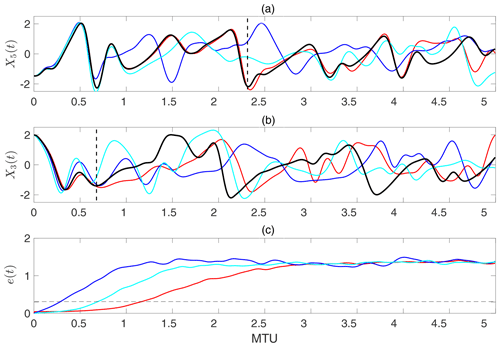Figure 5Comparison of the short-term forecasting skills among the three ML methods. The lines show truth (black), RC–ESN (red), ANN (blue), and RNN–LSTM (cyan). Panels (a) and (b) show examples (differing in initial condition) for which RC–ESN yields the longest and shortest prediction horizons, respectively. Vertical broken lines show the approximate prediction horizon of RC–ESN. Panel (c) shows the relative L2 error averaged over 100 randomly chosen initial conditions e(t); see Eq. 8. The broken horizontal line marks e=0.3. Time is in MTU, where $\mathrm{1}\phantom{\rule{0.125em}{0ex}}\mathrm{MTU}=\mathrm{200}\mathrm{\Delta }t\approx \mathrm{4.5}/{\mathit{\lambda }}_{max}$. Training size is N=500 000. ρ=0.1 is used here.

For the initial condition in Fig. 5a, RC–ESN accurately predicts the time series for over 2.3MTU, which is equivalent to 460Δt and over 10.35 Lyapunov timescales. Closer examination shows that the RC–RSN predictions follow the true trajectory well even up to ≈4MTU. The RNN–LSTM has the next-best prediction performance (up to around 0.9MTU or 180Δt). The predictions from ANN are around 0.6MTU or 120Δt. For the example in Fig. 5b, all methods have shorter prediction horizons, but RC–ESN still has the best performance (accurate predictions up to ≈0.7MTU), followed by ANN and RNN–LSTM, with similar prediction accuracies (≈0.3MTU). Searching through all 100 initial conditions, the best predictions with RNN–LSTM are up to ≈1.7MTU (equivalent to 340Δt), and the best predictions with ANN are up to ≈1.2MTU (equivalent to 240Δt).

To compare the results over all 100 randomly chosen initial conditions, we have defined an averaged relative L2 error between the true and predicted trajectories as follows:

$\begin{array}{}\text{(8)}& e\left(t\right)=\left[\frac{||{X}_{\text{true}}\left(t\right)-{X}_{\text{pred}}\left(t\right)||}{〈||{X}_{\text{true}}\left(t\right)||〉}\right].\end{array}$

Here [⋅] and 〈⋅〉 indicate, respectively, averaging over 100 initial conditions and over 2000Δt. To be clear, Xtrue(t) refers to the data at time t obtained from the numerical solution, while Xpred(t) refers to the predicted value at t using one of the machine-learning methods. Figure 5c compares e(t) for the three methods. It is clear that RC–ESN significantly outperforms ANN and RNN–LSTM for short-term predictions in this multiscale chaotic test bed. Figure 6 shows an example of the spatiotemporal evolution of Xpred (from RC–ESN), Xtrue, and their difference, which further demonstrates the capabilities of RC–ESN for short-term spatiotemporal predictions.

## 3.2 Short-term predictions: scaling of RC–ESN and ANN performance with training size N

How the performance of ML techniques scales with the size of the training set is of significant practical importance, as the amount of available data is often limited in many problems. Given that there is currently no theoretical understanding of such scaling for these ML techniques, we have empirically examined how the quality of short-term predictions scale with N. We have conducted the scaling analysis for N=104 to $N=\mathrm{2}×{\mathrm{10}}^{\mathrm{6}}$ for the three methods. Two metrics for the quality of predictions are used, namely the prediction horizon, defined as when the averaged L2 error e(t) reaches 0.3, and the prediction error E, defined as the average of e(t) between 0 and 0.5MTU as follows:

$\begin{array}{}\text{(9)}& E=\frac{\mathrm{1}}{\mathrm{100}\mathrm{\Delta }t}\sum _{i=\mathrm{0}}^{i=\mathrm{100}}e\left(i\mathrm{\Delta }t\right).\end{array}$

Figure 7a shows that, for all methods, the prediction horizon increases monotonically, but nonlinearly, as we increase N. The prediction horizons of RC–ESN and ANN appear to saturate after N=106, although the RC–ESN has a more complex, step-like scaling curve that needs further examination in future studies. The prediction horizon of RC–ESN exceeds that of ANN by factors ranging from 3 (for high N) to 9 (for low N). In the case of RNN–LSTM, the factor ranges from 1.2 (for high N) to 2 (for low N). Figure 7b shows that, for all methods, the average error E decreases as N increases (as expected), most notably for ANN when N is small.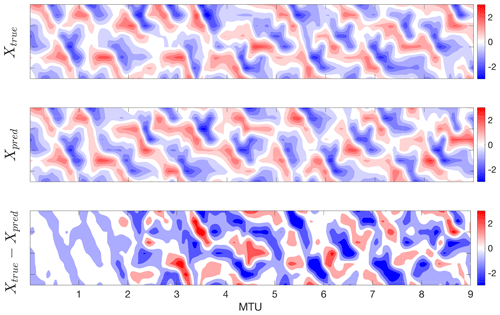Figure 6Performance of RC–ESN for short-term spatiotemporal predictions. We remind the readers that only the slow/large-scale variable X has been used during training/testing, and the fast/small-scale variables Y and Z have not been used at any point during training or testing. RC–ESN has substantial forecasting skills, providing accurate predictions up to ≈2MTU, which is around 400Δt or 9 Lyapunov timescales. N=500 000, algorithm T2, D=5000, and ρ=0.1 are used.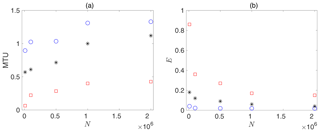Figure 7Comparison of the short-term predictions quality for RC–ESN (blue circles), RNN–LSTM (black stars), and ANN (red squares) as the size of the training set N is changed from N=104 to $N=\mathrm{2}×{\mathrm{10}}^{\mathrm{6}}$. Panel (a) shows the prediction horizon (when e(t) reaches 0.3), and panel (b) shows the average error E (see Eq. 9). The hyperparameters in each method are optimized for each N.

Overall, compared to both RNN–LSTM and ANN, the prediction horizon and accuracy of RC–ESN have a weaker dependence on the size of the training set, which is a significant advantage for RC–ESN when the dataset available for training is short, which is common in many practical problems.

## 3.3 Short-term predictions: scaling of RC–ESN performance with reservoir size D

Given the superior performance of RC–ESN for short-term predictions, here we focus on one concern with this method, namely the need for large reservoirs, which can be computationally demanding. This issue has been suggested as a potential disadvantage of ESNs versus LSTMs for training RNNs (Jaeger2007). Aside from the observation here that RC–ESN significantly outperforms RNN–LSTM for short-term predictions, the problem of reservoir size can be tackled in at least two ways. First, have proposed, and shown the effectiveness of, using a set of parallelized reservoirs, which allow one to easily deal with high-dimensional chaotic toy models.

Second, Fig. 8 shows that E rapidly declines by a factor of around 3 as D is increased from 500 to 5000, decreases slightly as D is further doubled to 10 000, and then barely changes as D is doubled again to 20 000. Training the RC–ESN with D=20 000 versus D=5000 comes with a higher computational cost (16 GB memory and ≈6 CPU hours for D=5000 and 64 GB memory and ≈18 CPU hours for D=20 000), while little improvement in accuracy is gained. Thus, concepts from inexact computing can be used to choose D so that precision is traded for large savings in computational resources, which can then be reinvested into more simulations, higher resolutions for critical processes, etc. .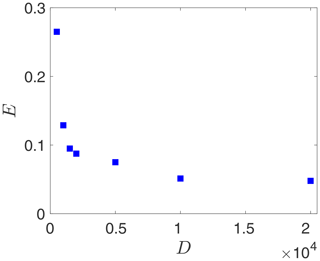Figure 8Scaling of average prediction error between 0 and 0.5MTU (E; Eq. 9) with reservoir size D for RC–ESN. N=500 000 is used.

## 3.4 Long-term statistics: comparison of RC–ESN, ANN, and RNN–LSTM performance

All the data-driven predictions discussed earlier eventually diverge from the true trajectory (as would even predictions using the numerical solver). Still, it is interesting to examine whether the freely predicted spatiotemporal data have the same long-term statistical properties as the actual dynamical system (i.e., Eqs. 13). Reproducing the actual dynamical system's long-term statistics (sometimes referred to as the system's climate) using a data-driven method can be significantly useful. In some problems, a surrogate model does not need to predict the evolution of a specific trajectory but only the long-term statistics of a system. Furthermore, synthetic long datasets produced using an inexpensive data-driven method (trained on a shorter real dataset) can be used to examine the system's probability density functions (pdf's), including its tails, which are important for studying the statistics of rare/extreme events.

By examining return maps, have already shown that RC–ESNs can reproduce the long-term statistics of the Lorenz 63 and Kuramoto–Sivashinsky equations. and have also shown that RC–ESNs can be used to accurately estimate a chaotic system's Lyapunov spectrum. Here, we focus on comparing the performance of RC–ESN, ANN, and RNN–LSTM in reproducing the system's long-term statistics by doing the following:

• examining the estimated pdf's and in particular their tails

• investigating whether the quality of the estimated pdf's degrades with time, which can negate the usefulness of long synthetic datasets.

Figure 9 compares the estimated pdf's obtained using the three ML methods. The data predicted using RC–ESN and RNN–LSTM are found to have pdf's closely matching the true pdf, even at the tails. Deviations at the tails of the pdf predicted by these methods from the true pdf are comparable to the deviations of the pdf's obtained from true data using the same number of samples. The ANN-predicted data have reasonable pdf's at ±2 standard deviation (SD), but the tails have substantial deviations from those of the true pdf's. All predicted pdf's are robust and do not differ much (except near the end of the tails) among the quartiles.

The results show that RC–ESN and RNN–LSTM can accurately reproduce the system's long-term statistics and can robustly produce long synthetic datasets with pdf's that are close to the pdf of the true data – even near the tails. The ability of ANN to accomplish these tasks is limited.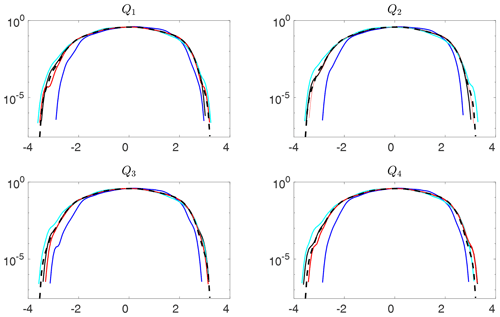Figure 9Estimated probability density functions (pdf's) of long datasets freely predicted by using RC–ESN (red), ANN (blue), and RNN–LSTM (cyan) compared to the true pdf (broken black). RC–ESN, ANN, and RNN–LSTM are first trained with N=500 000 and then predict, from an initial condition, for 4×106Δt. Panels Q1Q4 correspond to the equally divided quartiles of 106 predicted samples. The pdf's are approximated using kernel density estimation . The green lines show the estimated pdf's from different sets of 106 samples that are obtained from the numerical solution of Eqs. (1)–(3). Small differences between the green lines show the uncertainties in estimating the tails from 106 samples. The broken black lines show the true pdf estimated from 107 samples from the numerical solution. Note that the presented pdf's are for standardized data.

4 Discussion

By examining the true and predicted trajectories (Figs. 56) and the prediction errors and horizons (Fig. 7), we have shown that RC–ESN substantially outperforms ANN and RNN–LSTM in predicting the short-term evolution of a multiscale Lorenz 96 chaotic system (Eqs. 13). Additionally, RC–ESN and RNN–LSTM both work well in reproducing the long-term statistics of this system (Fig. 9). We emphasize that, following the problem formulation of , and unlike most other studies, only part of the multiscale state vector (the slow/large-scale variable X) has been available for training the data-driven model and has been of interest for testing. This problem design is more relevant to many practical problems but is more challenging as it requires the data-driven model to not only predict the evolution of X based on its past observations but also to account for the effects of the intermediate and fast/small-scale variables Y and Z on X.

We have also found that the prediction horizon of RC–ESN, and even more so its prediction accuracy, to weakly depend on the size of the training set (Fig. 7). This is an important property as in many practical problems the data available for training are limited. Furthermore, the prediction error of RC–ESN is shown to have an asymptotic behavior for large reservoir sizes, which suggests that reasonable accuracy can be achieved with moderate reservoir sizes. Note that the skillful predictions with RC–ESN in Figs. 56 have been obtained with a moderate-sized training set (N=500 000) and reservoir (D=5000). Figures 7 and 8 suggest that slightly better results could have been obtained using larger N and D, although such improvements come at a higher computational cost during training.

The order we have found for the performance of the three machine-learning methods (RC–ESN > RNN–LSTM > ANN) is different from the order of complexity of these methods in terms of the number of trainable parameters (RC–ESN < ANN < RNN–LSTM). But the order of performance is aligned with the order in terms of the learnable function space, namely ANN < RC–ESN and RNN–LSTM. The latter methods are both RNNs and thus Turing complete, while ANN is a stateless feed-forward network and thus not Turing complete . The Turing completeness of RNNs, in theory, enables RNNs to compute any function or implement any algorithm. Furthermore, state augmentation of ANN is not possible because it has no state. Whether the superior predictive performance of RC–ESN (especially over RNN–LSTM) is due to its explicit state representation, which is updated at every time step during both training and testing, or its lower likelihood of overfitting due to the order of magnitude having a smaller number of trainable parameters remains to be seen. Also, whether we have fully harnessed the power of RNN–LSTMs (see below) is unclear at this point, particularly because a complete theoretical understanding of how/why these methods work (or do not work) is currently lacking. That said, there has been some progress in understanding the RC–ESNs, in particular by modeling the network itself as a dynamical system . Such efforts, for example those aimed at understanding the echo states that are learned in the RC–ESN's reservoir, might benefit from recent advances in dynamical systems theory, e.g., for analyzing nonlinear spatiotemporal data .

An important next step in our work is to determine how generalizable our findings are. This investigation is important for the following two reasons. First, here we have only studied one system, which is a specially designed version of the Lorenz 96 system. The performance of these methods should be examined in a hierarchy of chaotic dynamical systems and high-dimensional turbulent flows. That said, our findings are overall consistent with the recently reported performance of these methods applied to chaotic toy models. It has been demonstrated that RC–ESN can predict, for several Lyapunov timescales, the spatiotemporal evolution and can reproduce the climate of the Lorenz 63 and Kuramoto–Sivashinsky chaotic systems . Here, we have shown that RC–ESN performs similarly well even when only the slow/large-scale component of the multiscale state vector is known. Our results with ANN are consistent with those of , who showed examples of trajectories predicted accurately up to 1MTU with a large training set ($N=\mathrm{2}×{\mathrm{10}}^{\mathrm{6}}$) and using ANN for the same Lorenz 96 system (see their Fig. 1). While RNN–LSTM is considered to be state of the art for sequential data modeling, and has worked well for a number of applications involving time series to the best of our knowledge, simple RNN–LSTMs, such as the one used here, have not been very successful when applied to chaotic dynamical systems. found some prediction skills, using RNN–LSTM applied to the original Lorenz 96 system, which does not have the multiscale coupling (see their Fig. 5).

Second, we have only used a simple RNN–LSTM. There are other variants of this architecture and more advanced deep-learning RNNs that might potentially yield better results. For our simple RNN–LSTM, we have extensively explored the optimization of hyperparameters and tried various formulations of the problem, e.g., predicting ΔX(t) with or without updating the state of the LSTM. We have found that such variants do not lead to better results, which is consistent with the findings of . Our preliminary explorations with more advanced variants of RNN–LSTM, e.g., seq2seq and encoder-decoder LSTM , have not resulted in any improvement either. However, just as the skillful predictions of RC–ESN and ANN hinge on one key step (nonlinear transformation for the former and predicting ΔX for the latter), it is possible that changing/adding one step leads to major improvements in RNN–LSTM. It is worth mentioning that similar nonlinear transformation of the state in LSTMs is not straightforward due to their more complex architecture; see Appendix D. We have shared our codes publicly to help others explore such changes to our RNN–LSTM. Furthermore, there are other, more sophisticated RNNs that we have not yet explored. For example, have introduced a tensor-train RNN that outperformed a simple RNN–LSTM in predicting the temporal evolution of the Lorenz 63 chaotic system. showed that a compressed convolutional LSTM performs well in capturing the long-term statistics of a turbulent flow. The performance of more advanced deep-learning RNNs should be examined and compared, side by side, with the performance of RC–ESNs and ANNs in future studies. The multiscale Lorenz 96 system provides a suitable starting point for such comparisons.

The results of our work show the promise of ML methods, such as RC–ESNs (and to some extent RNN–LSTM), for data-driven modeling of chaotic dynamical systems, which have broad applications in geosciences, e.g., in weather and climate modeling. Practical and fundamental issues such as interpretability; scalability to higher-dimensional systems ; presence of measurement noise in the training data and initial conditions ; nonstationarity of the time series; and dealing with data that have two or three spatial dimensions (e.g., through integration with convolutional neural networks, namely CNN–LSTM, and CNN–ESN should be studied in future work).

Here we have focused on a fully data-driven approach, as opposed to the conventional approach of the direct numerical solutions (Fig. 2). In practice, for example, for large-scale multiphysics, multiscale dynamical systems such as weather and climate models, it is likely that a hybrid framework yields the best performance. In such a framework, depending on the application and the spatiotemporal scales of the physical processes involved , some of the equations could be solved numerically with double precision, some could be solved numerically with lower precisions, and some could be approximated with a surrogate model learned via a data-driven approach, such as the ones studied in this paper.

Appendix A: More details on RC–ESN

Here we show a comparison of RC–ESN forecasting skills with nonlinear transformation algorithms T1 , T2, and T3 and without any transformation between r and $\stackrel{\mathrm{̃}}{\mathbf{r}}$. The three algorithms are (for $i=\mathrm{1},\mathrm{2},\mathrm{3}\mathrm{\dots }N$ and $j=\mathrm{1},\mathrm{2},\mathrm{3}\mathrm{\dots }D$) as follows:

Algorithm T1

Algorithm T2

Algorithm T3

Figure A1a shows an example of short-term predictions from an initial condition using T1, T2, T3, and no transformation; everything else was kept the same. It is clear that the nonlinear transformation is essential for skillful predictions, as the predictions obtained without transformation diverge from the truth before 0.25MTU. The three nonlinear transformation algorithms yield similar results, with accurate predictions for more than 2 MTU. Figure A1b, which shows the relative prediction error averaged over 100 initial conditions, further confirms this point.

Why the nonlinear transformation is needed, and the best/optimal choice for the transformation (if it exists), should be studied in future work. We highlight that the nonlinear transformation resembles basis function expansion, which is commonly used to capture nonlinearity in linear regression models (Bishop2006).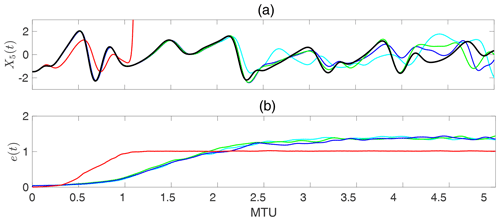Figure A1Comparison of RC–ESN forecasting skills with nonlinear transformation algorithms T1, T2, and T3 (cyan, green, and blue lines, respectively) and without any transformation (red line). Black line shows the truth. (a) Predictions from one initial condition and (b) relative L2-norm error averaged over 100 initial condition e(t) (Eq. 8). Time is in MTU, where $\mathrm{1}\phantom{\rule{0.125em}{0ex}}\mathrm{MTU}=\mathrm{200}\mathrm{\Delta }t\approx \mathrm{4.5}/{\mathit{\lambda }}_{max}$.

Appendix B: Dependence of RC–ESN's prediction horizon on ρ

We have conducted an extensive experimental analysis of the dependence of RC–ESN's prediction horizon on the value of ρ and found ρ=0.1 to produce the best prediction horizon for any sample size, N, and reservoir size, D, for this system. Table B1 shows part of the results obtained from the experiments with N=500 000, N=106, and with D=5000 over 100 initial conditions. While ρ=0.1 yields the best results, we see that the prediction horizon is barely sensitive to the choice of ρ within a wide range and similar overall prediction horizons are obtained for $\mathrm{0.05}\le \mathit{\rho }\le \mathrm{0.7}$.

Table B1Prediction horizon (MTU) over 100 initial conditions for an RC–ESN with samples sizes of N=106, N=500 000, and D=5000 for different ranges of ρ.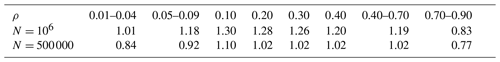Appendix C: More details on ANN

The ANN used in this study (and the one that yields the best performance) is similar to that of . The ANN has four hidden layers, each of which has 100 neurons with a tanh activation function. The input to the ANN has 8 neurons which takes (X(t))test as the input and outputs X(t))test which is also a 8-neuron layer. The obtained X(t))test output during the test is then used in an Adams–Bashforth integration scheme to obtain (X(tt))test. We found that a simpler Euler scheme yields similar results. However, we found that training/testing on pairs of X(t) and X(tt), which was used for RC–ESN, leads to no prediction skill with ANN, and that following the procedure used in is essential for skillful predictions. We speculate that this might be due to the stateless nature of the ANN. By relating X(t) to the change in X(t) at the next time step, rather than the raw value X(tt), this ANN training architecture implicitly contains a reference to the previous time step. While the ANN itself is stateless, this particular training approach essentially encodes a first-order temporal dependence between successive states.

Note that our approach here is the same as the global ANN of . We also tried their local ANN approach but, consistent with their findings for the Lorenz system, found better performance with the global approach (results not reported for brevity).

The ANN is trained with a stochastic gradient descent algorithm with a learning rate of 0.001, with a batch size of 100, and mean absolute error as the loss function (mean squared error also gives similar performance).

Appendix D: More details on RNN–LSTM

The governing equations for RNN–LSTM are as follows:

$\begin{array}{}\text{(D1)}& & {g}^{f}\left(t\right)={\mathit{\sigma }}_{f}\left({\mathbf{W}}_{\mathbf{f}}\left[h\left(t-\mathrm{1}\right),I\left(t\right)\right]+{b}_{f}\right),\text{(D2)}& & {g}^{i}\left(t\right)={\mathit{\sigma }}_{f}\left({\mathbf{W}}_{\mathbf{i}}\left[h\left(t-\mathrm{1}\right),I\left(t\right)\right]+{b}_{i}\right),\text{(D3)}& & \stackrel{\mathrm{̃}}{C}\left(t\right)=\mathrm{tanh}\left({\mathbf{W}}_{\mathbf{c}}\left[h\left(t-\mathrm{1}\right),I\left(t\right)\right]+{b}_{h}\right),\text{(D4)}& & C\left(t\right)={g}^{f}\left(t\right)C\left(t-\mathrm{1}\right)+{g}^{i}\left(t\right)\stackrel{\mathrm{̃}}{C}\left(t\right),\text{(D5)}& & {g}^{o}\left(t\right)={\mathit{\sigma }}_{h}\left({\mathbf{W}}_{\mathbf{h}}\left[h\left(t-\mathrm{1}\right),I\left(t\right)\right]+{b}_{h}\right),\text{(D6)}& & h\left(t\right)={g}^{o}\left(t\right)\mathrm{tanh}\left(C\left(t\right)\right),\text{(D7)}& & \mathrm{\Phi }\left(t\right)={\mathbf{W}}_{\mathbf{oh}}h\left(t\right),\text{(D8)}& & X\left(t+\mathrm{\Delta }t\right)\approx \mathrm{\Phi }\left(t\right).\end{array}$

σf is the softmax activation function and gf(t), gi(t), and go(t) $\in {\mathrm{\Re }}^{{d}_{h}×\left({d}_{h}+{d}_{i}\right)}$ are the forget gate, input gate, and output gate, respectively. dh is the dimension of the hidden layers (chosen as 50 in our study), and di is the dimension of the input (q). bf, bi, and bh are the biases in the forget gate, input gate, and the hidden layers. $I\left(t\right)\in {\mathrm{\Re }}^{{d}_{i}}$ is the input, which is a column of a time-delay-embedded matrix of X(t). This matrix has the dimension of $\left(\mathrm{8}×q\right)×N$. $h\left(t\right)\in {\mathrm{\Re }}^{{d}_{h}}$ is the hidden state and $C\left(t\right)\in {\mathrm{\Re }}^{{d}_{h}}$ is the cell state (the states track the temporal evolution). The weights Wo, Wi, Wf, Wc, and Woh are learned through the BPTT algorithm using an Adam optimizer . Φ(t) is the output from the RNN–LSTM.

The LSTM used in this study is a stateless LSTM, in which the two hidden states (C and h) are refreshed at the beginning of each batch during training (this is not the same as the stateless ANN, where there is no hidden state at all). Here, the training sequences in each batch are shuffled randomly, leading to an unbiased gradient estimator in the stochastic gradient descent algorithm .

Code and data availability

All data and codes can be accessed at https://github.com/ashesh6810/RCESN_spatio_temporal ().

Author contributions

All authors developed the idea. PH designed and supervised the study. AC, with help from DS, developed the machine-learning codes. All authors discussed the results and revised the paper.

Competing interests

The authors declare that they have no conflict of interest.

Acknowledgements

Computational resources on Bridges Large, Bridges GPU, and Stampede2 clusters were provided by the National Science Foundation (NSF) XSEDE allocation (grant no. ATM170020). We are grateful to Krishna Palem for many stimulating discussions and helpful comments. We thank Michelle Girvan, Reza Malek-Madani, Rohan Mukherjee, Guido Novati, and Pantelis Vlachas for the insightful discussions. We thank Jaideep Pathak for sharing his MATLAB ESN code for the Kuramoto–Sivashinsky system. We are grateful to Mingchao Jiang and Adam Subel for their help with running some of the simulations. We also thank the editor, Zoltan Toth, and Istvan Szunyogh and an anonymous reviewer for providing valuable feedback.

Financial support

This research has been supported, through funding given to Pedram Hassanzadeh, by the National Aeronautics and Space Administration (grant no. 80NSSC17K0266) and the Gulf Research Program (Early-Career Research Fellowship from the National Academies of Science, Engineering, and Medicine). Ashesh Chattopadhyay has been supported by the Ken Kennedy Institute at Rice University for a BP HPC Graduate Fellowship.

Review statement

This paper was edited by Zoltan Toth and reviewed by Istvan Szunyogh and one anonymous referee.

References

Andersen, J. and Kuang, Z.: Moist static energy budget of MJO-like disturbances in the atmosphere of a zonally symmetric aquaplanet, J. Climate, 25, 2782–2804, 2012. a

Arbabi, H. and Mezic, I.: Ergodic theory, dynamic mode decomposition, and computation of spectral properties of the Koopman operator, SIAM J. Appl. Dynam. Syst., 16, 2096–2126, 2017. a

Bauer, P., Thorpe, A., and Brunet, G.: The quiet revolution of numerical weather prediction, Nature, 525, 47–55, https://doi.org/10.1038/nature14956, 2015. a

Benedict, J. and Randall, D.: Structure of the Madden–Julian oscillation in the superparameterized CAM, J. Atmos. Sci., 66, 3277–3296, 2009. a

Bishop, C.: Pattern Recognition and Machine Learning, Springer, 2006. a

Bolton, T. and Zanna, L.: Applications of deep learning to ocean data inference and subgrid parameterization, J. Adv. Model. Earth Sy., 11, 376–399, 2019. a

Brenowitz, N. and Bretherton, C.: Prognostic validation of a neural network unified physics parameterization, Geophys. Res. Lett., 45, 6289–6298, 2018. a

Brunton, S. and Kutz, J.: Data-driven Science and Engineering: Machine Learning, Dynamical Systems, and Control, Cambridge University Press, 2019. a

Carbonneau, R., Laframboise, K., and Vahidov, R.: Application of machine learning techniques for supply chain demand forecasting, Eur. J. Operat. Res., 184, 1140–1154, 2008. a

Chantry, M., Thornes, T., Palmer, T., and Düben, P.: Scale-selective precision for weather and climate forecasting, Mon. Weather Rev., 147, 645–655, 2019. a

Chattopadhya, A.: RC_ESN_spatio_temporal, available at: https://github.com/ashesh6810/RCESN_spatio_temporal, GitHub, last access: 29 June 2020. a

Chattopadhyay, A., Hassanzadeh, P., and Pasha, S.: Predicting clustered weather patterns: A test case for applications of convolutional neural networks to spatio-temporal climate data, Sci. Rep., 10, 1–13, 2020a. a

Chattopadhyay, A., Nabizadeh, E., and Hassanzadeh, P.: Analog forecasting of extreme-causing weather patterns using deep learning, J. Adv. Model. Earth Sy., 12, https://doi.org/10.1029/2019MS001958, 2020b. a

Chattopadhyay, A., Subel, A., and Hassanzadeh, P.: Data-driven super-parameterization using deep learning: Experimentation with multi-scale Lorenz 96 systems and transfer-learning, arXiv [preprint], arXiv:2002.11167, 25 February 2020c. a

Chen, K., Zhou, Y., and Dai, F.: A LSTM-based method for stock returns prediction: A case study of China stock market, in: 2015 IEEE International Conference on Big Data, 29 October–1 November, Santa Clara, CA, USA, 2823–2824, IEEE, 2015. a

Cho, K., V.M, B., Gulcehre, C., Bahdanau, D., Bougares, F., Schwenk, H., and Bengio, Y.: Learning phrase representations using RNN encoder-decoder for statistical machine translation, arXiv [preprint], arXiv:1406.1078, 2014. a

Collins, W., Rasch, P., Boville, B., Hack, J., McCaa, J., Williamson, D., Briegleb, B., Bitz, C., Lin, S., and Zhang, M.: The formulation and atmospheric simulation of the Community Atmosphere Model version 3 (CAM3), J. Climate, 19, 2144–2161, 2006. a

Collins, W. J., Bellouin, N., Doutriaux-Boucher, M., Gedney, N., Halloran, P., Hinton, T., Hughes, J., Jones, C. D., Joshi, M., Liddicoat, S., Martin, G., O'Connor, F., Rae, J., Senior, C., Sitch, S., Totterdell, I., Wiltshire, A., and Woodward, S.: Development and evaluation of an Earth-System model – HadGEM2, Geosci. Model Dev., 4, 1051–1075, https://doi.org/10.5194/gmd-4-1051-2011, 2011. a

Düben, P. and Palmer, T.: Benchmark tests for numerical weather forecasts on inexact hardware, Mon. Weather Rev., 142, 3809–3829, 2014. a, b

Düben, P., Joven, J., Lingamneni, A., McNamara, H., De Micheli, G., Palem, K., and Palmer, T.: On the use of inexact, pruned hardware in atmospheric modelling, Philos. T. Roy. Soc. A-Math., 372, 1–16, https://doi.org/10.1098/rsta.2013.0276, 2014. a

Düben, P., Yenugula, S., Augustine, J., Palem, K., Schlachter, J., Enz, C., and Palmer, T.: Opportunities for energy efficient computing: A study of inexact general purpose processors for high-performance and big-data applications, in: 2015 Design, Automation and Test in Europe Conference and Exhibition (DATE), 9–12 March, Grenoble, France, 764–769, IEEE, 2015. a

Dueben, P. D. and Bauer, P.: Challenges and design choices for global weather and climate models based on machine learning, Geosci. Model Dev., 11, 3999–4009, https://doi.org/10.5194/gmd-11-3999-2018, 2018. a, b, c, d, e, f, g, h, i, j, k

Duraisamy, K., Iaccarino, G., and Xiao, H.: Turbulence modeling in the age of data, Ann. Rev. Fluid Mech., 51, 357–377, 2019. a

Epanechnikov, V.: Non-parametric estimation of a multivariate probability density, Theor. Prob. Appl., 14, 153–158, 1969. a

Fan, H., Jiang, J., Zhang, C., Wang, X., and Lai, Y.-C.: Long-term prediction of chaotic systems with machine learning, Phys. Rev. Res., 2, 012080, https://doi.org/10.1103/PhysRevResearch.2.012080, 2020. a

Flato, G.: Earth system models: an overview, WIRES Clim. Change, 2, 783–800, 2011. a

Gagne, I., John, D., Christensen, H. M., Subramanian, A. C., and Monahan, A. H.: Machine Learning for Stochastic Parameterization: Generative Adversarial Networks in the Lorenz'96 Model, J. Adv. Model. Earth Sy., 12, https://doi.org/10.1029/2019MS001896, 2020. a

Garcia, R., Smith, A., Kinnison, D., Cámara, Á. l., and Murphy, D.: Modification of the gravity wave parameterization in the Whole Atmosphere Community Climate Model: Motivation and results, J. Atmos. Sci., 74, 275–291, 2017. a

Gauthier, D.: Reservoir computing: Harnessing a universal dynamical system, SIAM News, 51, 12, 2018. a, b

Gentine, P., Pritchard, M., Rasp, S., Reinaudi, G., and Yacalis, G.: Could machine learning break the convection parameterization deadlock?, Geophys. Res. Lett., 45, 5742–5751, 2018. a

Giannakis, D., Ourmazd, A., Slawinska, J., and Zhao, Z.: Spatiotemporal pattern extraction by spectral analysis of vector-valued observables, arXiv preprint arXiv:1711.02798, 2017. a

Goodfellow, I., Bengio, Y., and Courville, A.: Deep learning, MIT Press, 2016. a, b, c, d

Graves, A., Mohamed, A., and Hinton, G.: Speech recognition with deep recurrent neural networks, in: 2013 IEEE international Conference on Acoustics, Speech and Signal Processing, 26–31 May, Vancouver, Canada, 6645–6649, IEEE, 2013. a

Hatfield, S., Subramanian, A., Palmer, T., and Düben, P.: Improving weather forecast skill through reduced-precision data assimilation, Mon. Weather Rev., 146, 49–62, 2018. a

Hochreiter, S. and Schmidhuber, J.: Long short-term memory, Neural Comput., 9, 1735–1780, 1997. a

Hourdin, F., M., T., G., A., Golaz, J., Balaji, V., Duan, Q., Folini, D., Ji, D., Klocke, D., Qian, Y., et al.: The art and science of climate model tuning, B. Am. Meteorol. Soc., 98, 589–602, 2017. a

Jaeger, H.: Echo state network, Scholarpedia, 2, 2330, https://doi.org/10.4249/scholarpedia.2330, revision #188245, 2007. a, b, c

Jaeger, H. and Haas, H.: Harnessing nonlinearity: Predicting chaotic systems and saving energy in wireless communication, Science, 304, 78–80, 2004. a, b, c

Jeevanjee, N., Hassanzadeh, P., Hill, S., and Sheshadri, A.: A perspective on climate model hierarchies, J. Adv. Model. Earth Sy., 9, 1760–1771, 2017. a, b

Khairoutdinov, M. and Randall, D.: A cloud resolving model as a cloud parameterization in the NCAR Community Climate System Model: Preliminary results, Geophys. Res. Lett., 28, 3617–3620, 2001. a

Khodkar, M. and Hassanzadeh, P.: Data-driven reduced modelling of turbulent Rayleigh–Bénard convection using DMD-enhanced fluctuation–dissipation theorem, J. Fluid Mech., 852, https://doi.org/10.1017/jfm.2018.586, 2018. a

Khodkar, M., Hassanzadeh, P., Nabi, S., and Grover, P.: Reduced-order modeling of fully turbulent buoyancy-driven flows using the Green's function method, Phys. Rev. Fluids, 4, 013801, https://doi.org/10.1103/PhysRevFluids.4.013801, 2019. a

Kim, H., Eykholt, R., and Salas, J.: Nonlinear dynamics, delay times, and embedding windows, Phys. D: Nonlin. Phenom., 127, 48–60, 1999. a

Kingma, D. and Ba, J.: Adam: A method for stochastic optimization, arXiv [preprint], arXiv:1412.6980, 22 December 2014. a

Kooperman, G., Pritchard, M., O'Brien, T., and Timmermans, B.: Rainfall From Resolved Rather Than Parameterized Processes Better Represents the Present-Day and Climate Change Response of Moderate Rates in the Community Atmosphere Model, J. Adv. Model. Earth Sy., 10, 971–988, 2018. a

Kutz, J.: Deep learning in fluid dynamics, J. Fluid Mech., 814, 1–4, 2017. a

Leyffer, S., Wild, S., Fagan, M., Snir, M., Palem, K., Yoshii, K., and Finkel, H.: Doing Moore with Less–Leapfrogging Moore's Law with Inexactness for Supercomputing, arXiv [preprint], arXiv:1610.02606, 9 October 2016. a, b

Lim, S. H., Giorgini, L. T., Moon, W., and Wettlaufer, J.: Predicting Rare Events in Multiscale Dynamical Systems using Machine Learning, arXiv [preprint], arXiv:1908.03771, 10 August 2019. a, b

Ling, J., Kurzawski, A., and Templeton, J.: Reynolds averaged turbulence modelling using deep neural networks with embedded invariance, J. Fluid Mech., 807, 155–166, 2016. a

Lorenz, E.: Predictability: A problem partly solved, in: Predcitibility of Weather and Climate, vol. 1, 40–58, ECMWF, 1996. a

Lu, Z., Pathak, J., Hunt, B., Girvan, M., Brockett, R., and Ott, E.: Reservoir observers: Model-free inference of unmeasured variables in chaotic systems, Chaos, 27, 041102, https://doi.org/10.1063/1.4979665, 2017. a, b, c

Lu, Z., Hunt, B., and Ott, E.: Attractor reconstruction by machine learning, Chaos, 28, 061104, https://doi.org/10.1063/1.5039508, 2018. a

Lukoševičius, M. and Jaeger, H.: Reservoir computing approaches to recurrent neural network training, Comput. Sci. Rev., 3, 127–149, 2009. a

Ma, Q., Shen, L., Chen, E., Tian, S., Wang, J., and Cottrell, G.: WALKING WALKing walking: Action Recognition from Action Echoes, in: Internationa Joint Conference on Artificial Intelligence, 19–25 August, Melbourne, Australia, 2457–2463, 2017. a

McDermott, P. and Wikle, C.: An ensemble quadratic echo state network for non-linear spatio-temporal forecasting, Stat, 6, 315–330, 2017. a, b

McDermott, P. and Wikle, C.: Deep echo state networks with uncertainty quantification for spatio-temporal forecasting, Environmetrics, 30, e2553, https://doi.org/10.1002/env.2553, 2019. a, b

Meng, Q., Chen, W., Wang, Y., Ma, Z.-M., and Liu, T.-Y.: Convergence analysis of distributed stochastic gradient descent with shuffling, Neurocomputing, 337, 46–57, 2019. a

Mezić, I.: Spectral properties of dynamical systems, model reduction and decompositions, Nonlin. Dynam., 41, 309–325, 2005. a

Mohan, A., Daniel, D., Chertkov, M., and Livescu, D.: Compressed Convolutional LSTM: An Efficient Deep Learning framework to Model High Fidelity 3D Turbulence, arXiv [preprint], arXiv:1903.00033, 28 February 2019. a, b

Moosavi, A., Attia, A., and Sandu, A.: A machine learning approach to adaptive covariance localization, arXiv [preprint], arXiv:1801.00548, 2 January 2018. a

O'Gorman, P. and Dwyer, J.: Using Machine Learning to Parameterize Moist Convection: Potential for Modeling of Climate, Climate Change, and Extreme Events, J. Adv. Model. Earth Sy., 10, 2548–2563, 2018. a

Palem, K.: Inexactness and a future of computing, Philos. T. Roy. Soc. A-Math., 372, 20130281, https://doi.org/10.1098/rsta.2019.0061, 2014. a, b, c

Palmer, T.: Climate forecasting: Build high-resolution global climate models, Nature News, 515, 338–339, https://doi.org/10.1038/515338a, 2014. a, b

Pascanu, R., Mikolov, T., and Bengio, Y.: On the difficulty of training recurrent neural networks, in: International Conference on Machine Learning, 16–21 June, Atlanta, USA, 1310–1318, 2013. a, b

Pathak, J., Lu, Z., Hunt, B., Girvan, M., and Ott, E.: Using machine learning to replicate chaotic attractors and calculate Lyapunov exponents from data, Chaos, 27, 121102, https://doi.org/10.1063/1.5010300, 2017. a, b, c, d, e

Pathak, J., Hunt, B., Girvan, M., Lu, Z., and Ott, E.: Model-free prediction of large spatiotemporally chaotic systems from data: A reservoir computing approach, Phys. Rev. Lett., 27, https://doi.org/10.1063/1.5010300, 2018a. a, b, c, d, e, f, g, h, i, j

Pathak, J., Wikner, A., Fussell, R., Chandra, S., Hunt, B., Girvan, M., and Ott, E.: Hybrid forecasting of chaotic processes: Using machine learning in conjunction with a knowledge-based model, Chaos, 28, 041101, https://doi.org/10.1063/1.5028373, 2018b. a

Raissi, M., Perdikaris, P., and Karniadakis, G.: Physics-informed neural networks: A deep learning framework for solving forward and inverse problems involving nonlinear partial differential equations, J. Comput. Phys., 378, 686–707, 2019. a

Rasp, S., Pritchard, M., and Gentine, P.: Deep learning to represent subgrid processes in climate models, P. Natl. Acad. Sci. USA, 115, 9684–9689, 2018. a

Reichstein, M., Camps-Vallis, G., Stevens, B., Jung, M., Denzler, J., and Prabhat, N. C.: Deep learning and process understanding for data-driven Earth system science, Nature, 566, 195–204, https://doi.org/10.1038/s41586-019-0912-1, 2019. a, b

Rudy, S., Kutz, J., and Brunton, S.: Deep learning of dynamics and signal-noise decomposition with time-stepping constraints, arXiv [preprint], arXiv:1808.02578, 7 August 2018. a, b

Salehipour, H. and Peltier, W.: Deep learning of mixing by two atoms of stratified turbulence, J. Fluid Mech., 861, https://doi.org/10.1017/jfm.2018.980, 2019. a

Scher, S. and Messori, G.: Generalization properties of feed-forward neural networks trained on Lorenz systems, Nonlin. Processes Geophys., 26, 381–399, https://doi.org/10.5194/npg-26-381-2019, 2019. a

Schneider, T., Lan, S., Stuart, A., and Teixeira, J.: Earth system modeling 2.0: A blueprint for models that learn from observations and targeted high-resolution simulations, Geophys. Res. Lett., 44, 12396–12417, https://doi.org/10.1002/2017GL076101, 2017a. a, b

Schneider, T., Teixeira, J., Bretherton, C., Brient, F., Pressel, K., Schär, C., and Siebesma, A.: Climate goals and computing the future of clouds, Nat. Clim. Change, 7, 3–5, https://doi.org/10.1038/nclimate3190, 2017b. a

Siegelmann, H. T. and Sontag, E. D.: On the computational power of neural nets, in: Proceedings of the fifth annual workshop on Computational learning theory, July, Pittsburgh, Pennsylvania, USA, 440–449, 1992. a

Stevens, B. and Bony, S.: What are climate models missing?, Science, 340, 1053–1054, 2013. a

Sutskever, I., Vinyals, O., and Le, Q.: Sequence to sequence learning with neural networks, in: Advances in Neural Information Processing Systems, 8–13 December, Montreal, Canada, 3104–3112, 2014. a

Thornes, T., Düben, P., and Palmer, T.: On the use of scale-dependent precision in Earth System modelling, Q. J. Roy. Meteorol. Soc., 143, 897–908, 2017. a, b, c, d, e, f

Toms, B. A., Kashinath, K., Yang, D., et al.: Deep Learning for Scientific Inference from Geophysical Data: The Madden–Julian oscillation as a Test Case, arXiv [preprint], arXiv:1902.04621, 12 February 2019. a

Tu, J., Rowley, C., Luchtenburg, D., Brunton, S., and Kutz, J.: On dynamic mode decomposition: Theory and applications, J. Comput. Dynam., 1, 391–421, 2014. a

Vlachas, P., Byeon, W., Wan, Z., Sapsis, T., and Koumoutsakos, P.: Data-driven forecasting of high-dimensional chaotic systems with long short-term memory networks, P. Roy. Soc. A-Math., 474, 20170844, https://doi.org/10.1098/rspa.2017.0844, 2018. a, b, c, d, e

Watson, P.: Applying machine learning to improve simulations of a chaotic dynamical system using empirical error correction, J. Adv. Model. Earth Sy., 11, 1402–1417, https://doi.org/10.1029/2018MS001597, 2019. a

Williams, M., Kevrekidis, I., and Rowley, C.: A data–driven approximation of the Koopman operator: Extending dynamic mode decomposition, J. Nonlin. Sci., 25, 1307–1346, 2015. a

Wu, J. et al.: Enforcing Statistical Constraints in Generative Adversarial Networks for Modeling Chaotic Dynamical Systems, arXiv [preprint], arXiv:1905.06841, 13 May 2019. a, b

Xingjian, S., Chen, Z., Wang, H., Yeung, D., Wong, W., and Woo, W.: Convolutional LSTM network: A machine learning approach for precipitation nowcasting, in: Advances in Neural Information Processing Systems, 7–12 December, Montreal, Canada, 802–810, 2015. a

Yildiz, I., Jaeger, H., and Kiebel, S.: Re-visiting the echo state property, Neural Networks, 35, 1–9, 2012. a

Yu, R., Zheng, S., Anandkumar, A., and Yue, Y.: Long-term forecasting using tensor-train RNNs, arXiv [preprint], arXiv:1711.00073, 31 October 2017. a

Zhu, Y., Zabaras, N., Koutsourelakis, P., and Perdikaris, P.: Physics-Constrained Deep Learning for High-dimensional Surrogate Modeling and Uncertainty Quantification without Labeled Data, arXiv [preprint], arXiv:1901.06314, 18 January 2019. a

Zimmermann, R. and Parlitz, U.: Observing spatio-temporal dynamics of excitable media using reservoir computing, Chaos, 28, 043118, https://doi.org/10.1063/1.5022276, 2018. a